### Home > CALC > Chapter 4 > Lesson 4.1.3 > Problem4-31

4-31.
1. Differentiate the following with respect to x. That is, find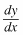. Homework Help ✎

1.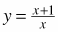2. y = cos x + sin x

3.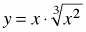4. y = (6 − 5x)(l − 2x)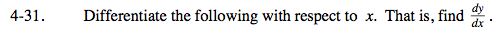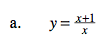Simplify the fraction:

$y=1+\frac{1}{x}$

= 1 + x −1

Differentiate:

$\frac{dy}{dx}=-x^{-2}$

$=-\frac{1}{x^{2}}$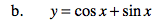Be careful about + and − signs.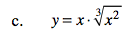Before you differentiate, rewrite with exponents.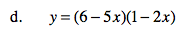Before you differentiate, expand.